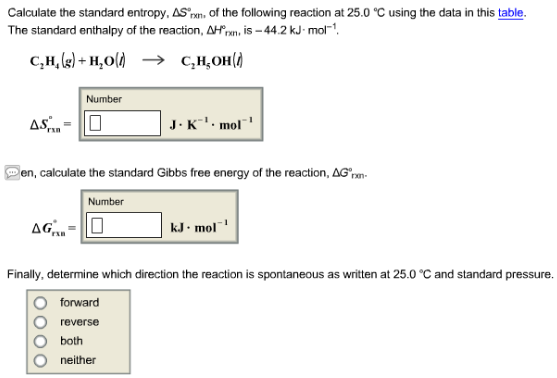# Problem: Calculate the standard entropy, ΔS°rxn, of the following reaction at 25.0 °C using the data in this table. The standard enthalpy of the reaction, ΔH°rxn is -44.2 kJ • mol-1 C2H4 (g) + H2O (l) → C2H5OH (l) Then, calculate the standard Gibbs free energy of the reaction, ΔG°rxn. Finally, determine which direction the reaction is spontaneous as written at 25.0 °C and standard pressure.

###### FREE Expert Solution
99% (114 ratings)###### Problem Details

Calculate the standard entropy, ΔS°rxn, of the following reaction at 25.0 °C using the data in this table.

The standard enthalpy of the reaction, ΔH°rxn is -44.2 kJ • mol-1

C2H4 (g) + H2O (l) → C2H5OH (l)

Then, calculate the standard Gibbs free energy of the reaction, ΔG°rxn

Finally, determine which direction the reaction is spontaneous as written at 25.0 °C and standard pressure.